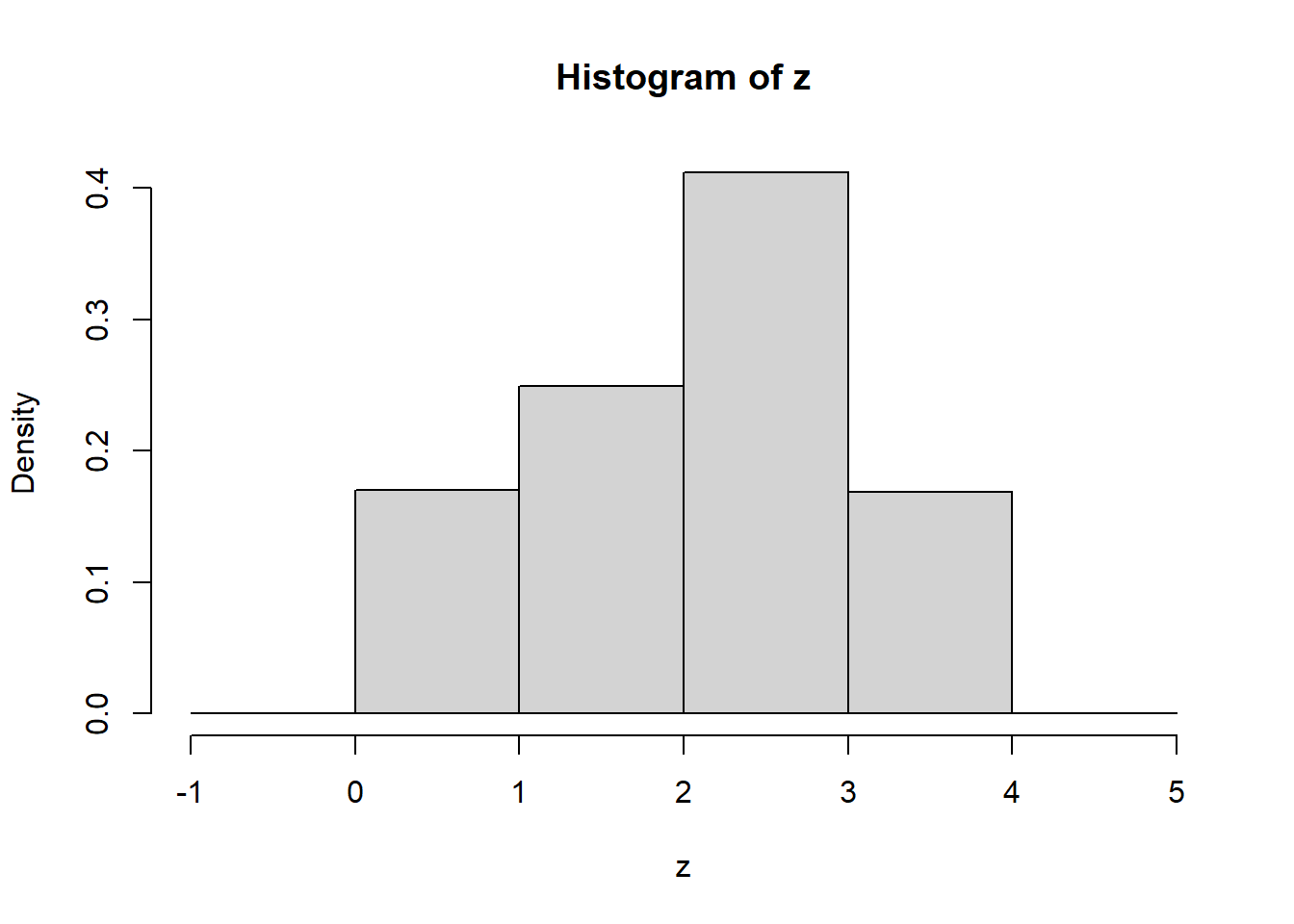In this worksheet, we will consider the output of a Markov chain that is stored on a textfile. We will import this data, and do basics statistics to estimate the stationary distribution of corresponding Markov chain.

• Suppose you are given the output of a $$100000$$ steps of a irreducible and aperiodic finite state Markov chain. Carefully explain how you could estimate the stationary distribution for this Markov chain, and why you estimator is reasonable.

• Import the data from the file markovchain.txt and use this data and your method above to estimate the stationary distribution.

• Any ideas for the transition matrix?

# Solutions

• Since the chain is on a finite state space and irreducible and aperiodic, it has a unique stationary distribution $$\pi$$, and we know that a version of the law of large numbers gives that for every state $$s$$, we have

$\frac{1}{n} \sum_{i=1}^n \mathbf{1}[X_i = s] \to \pi(s).$ Thus for each state, we simply need to count the number of occurrences and divide by $$100000$$, to get its approximate probability under $$\pi$$.

• A quick scan of the file shows there are $$4$$ states: $$1,2,3,4$$. With R, we have:
z =read.table("markovchain.txt", sep=",")
z= z[,]

b1 = seq(-1,5, by=1)
hist(z, prob=TRUE, breaks=b1)sum(z==1)/100000
##  0.17023
sum(z==2)/100000
##  0.24957
sum(z==3)/100000
##  0.41172
sum(z==4)/100000
##  0.16849

Next we show how this code might be done in Python; you may need to install the package pandas; if you are running this inside R, you may need to put py_install(“pandas”) where you load the library(reticulate).

import pandas as pd
import numpy as np

print(mydata)
##         x
## 1       1
## 2       2
## 3       4
## 4       3
## 5       4
## ...    ..
## 99997   3
## 99998   3
## 99999   2
## 100000  4
## 100001  3
##
## [100001 rows x 1 columns]
y = mydata.loc[:, 'x']
print(y)
## 1         1
## 2         2
## 3         4
## 4         3
## 5         4
##          ..
## 99997     3
## 99998     3
## 99999     2
## 100000    4
## 100001    3
## Name: x, Length: 100001, dtype: int64
print(np.mean(y==1))
## 0.17022829771702283
print(np.mean(y==2))
## 0.24956750432495675
print(np.mean(y==3))
## 0.4117158828411716
print(np.mean(y==4))

## 0.16848831511684884